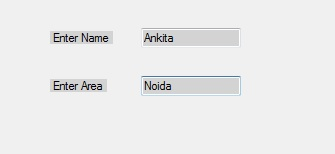Related Articles

# How to set the Margin between the TextBox controls in C#?

• Last Updated : 29 Nov, 2019

In Windows forms, Textbox plays an important role. With the help of TextBox, the user can enter data in the application, it can be of a single line or of multiple lines. In TextBox, you are allowed to set the space between two or more TextBox controls with the help of Margin Property. In Windows form, you can set this property in two different ways:

1. Design-Time: It is the simplest way to set the Margin property of the TextBox as shown in the following steps:

• Step 1: Create a windows form.
Visual Studio -> File -> New -> Project -> WindowsFormApp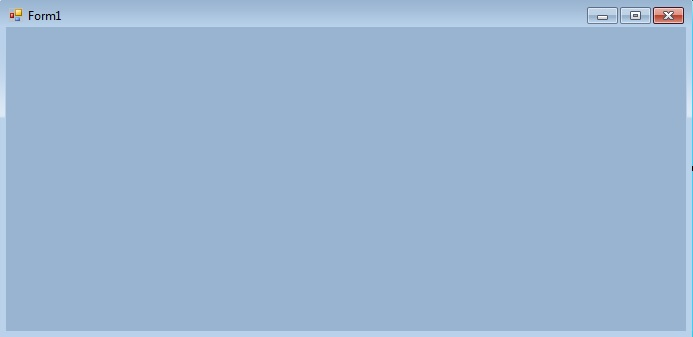• Step 2: Drag the TextBox control from the ToolBox and drop it on the windows form. You can place TextBox anywhere on the windows form according to your need.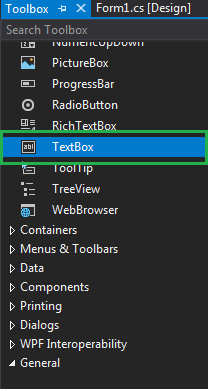• Step 3: After drag and drop you will go to the properties of the TextBox control to set the Margin property of the TextBox.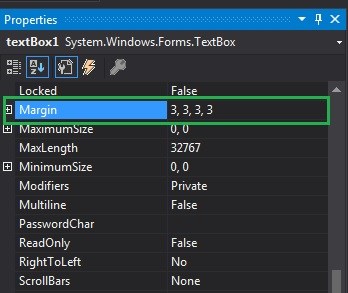Output: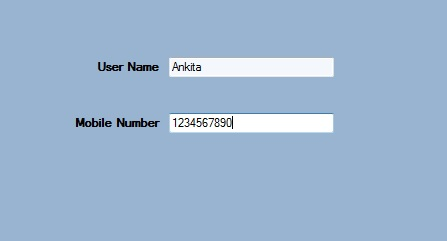2. Run-Time: It is a little bit trickier than the above method. In this method, you can set the Margin property of the TextBox programmatically with the help of given syntax:

`public System.Windows.Forms.Padding Margin { get; set; }`

Here, Padding is used to represent the space between the TextBox controls. Following steps are used to set the Margin property of the TextBox:

• Step 1 : Create a textbox using the TextBox() constructor provided by the TextBox class.
```// Creating textbox
TextBox Mytextbox = new TextBox();
```
• Step 2 : After creating TextBox, set the Margin property of the TextBox provided by the TextBox class.
```// Set Margin property
Mytextbox1.Margin = new Padding(5, 5, 5, 5);
```
• Step 3 : And last add this textbox control to from using Add() method.
```// Add this textbox to form
 `using` `System;``using` `System.Collections.Generic;``using` `System.ComponentModel;``using` `System.Data;``using` `System.Drawing;``using` `System.Linq;``using` `System.Text;``using` `System.Threading.Tasks;``using` `System.Windows.Forms;`` ` `namespace` `my {`` ` `public` `partial` `class` `Form1 : Form {`` ` `    ``public` `Form1()``    ``{``        ``InitializeComponent();``    ``}`` ` `    ``private` `void` `Form1_Load(``object` `sender, EventArgs e)``    ``{`` ` `        ``// Creating and setting the properties of Lable1``        ``Label Mylablel1 = ``new` `Label();``        ``Mylablel1.Location = ``new` `Point(96, 54);``        ``Mylablel1.Text = ``"Enter Name"``;``        ``Mylablel1.AutoSize = ``true``;``        ``Mylablel1.BackColor = Color.LightGray;`` ` `        ``// Add this label to form``        ``this``.Controls.Add(Mylablel1);`` ` `        ``// Creating and setting the properties of TextBox1``        ``TextBox Mytextbox1 = ``new` `TextBox();``        ``Mytextbox1.Location = ``new` `Point(187, 51);``        ``Mytextbox1.BackColor = Color.LightGray;``        ``Mytextbox1.AutoSize = ``true``;``        ``Mytextbox1.Name = ``"text_box1"``;``        ``Mytextbox1.Margin = ``new` `Padding(5, 5, 5, 5);`` ` `        ``// Add this textbox to form``        ``this``.Controls.Add(Mytextbox1);`` ` `        ``// Creating and setting the properties of Lable1``        ``Label Mylablel2 = ``new` `Label();``        ``Mylablel2.Location = ``new` `Point(96, 102);``        ``Mylablel2.Text = ``"Enter Area"``;``        ``Mylablel2.AutoSize = ``true``;``        ``Mylablel2.BackColor = Color.LightGray;`` ` `        ``// Add this label to form``        ``this``.Controls.Add(Mylablel2);`` ` `        ``// Creating and setting the properties of TextBox2``        ``TextBox Mytextbox2 = ``new` `TextBox();``        ``Mytextbox2.Location = ``new` `Point(187, 99);``        ``Mytextbox2.BackColor = Color.LightGray;``        ``Mytextbox2.AutoSize = ``true``;``        ``Mytextbox2.Name = ``"text_box2"``;`` ` `        ``// Add this textbox to form``        ``this``.Controls.Add(Mytextbox2);``    ``}``}``}`